# How to Subtract Cells in Excel

Feb 06, 2022

It’s no secret that Excel has the capability to perform hundreds of calculations at a time, ranging from basic computations to complex statistical functions. Excel uses cell references in its formulas so you can automate these functions with ease. For young professionals, these basic skills mean landing the job, getting the promotion, or maybe just having an easier time at work. This article will teach you how to perform subtraction in Microsoft Excel in three ways:

##The First Way: Subtracting cell values

Step one:

To get things started we want to obviously open Excel. We can do that by double-clicking on the desktop icon.Note that if you want to use an existing excel document, double-click on the excel document you need to open. (You can also do this in the file tab in Excel)

###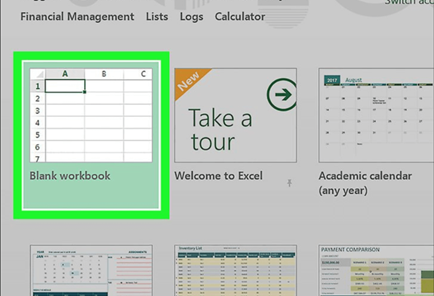Click "Blank workbook" (available on Windows) or "Excel workbook" (available on Mac). The button is at the top left of the Template window.

### Step Three: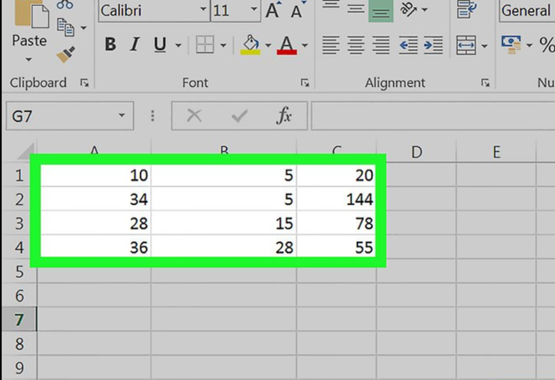Enter the data if needed. Do this by clicking on a cell, typing a number, and pressing ↵ Enter or ⏎ Return.

### Step Four: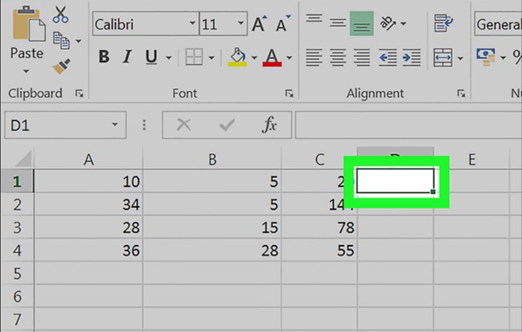Click on an empty cell. This selects the cell.

### Step Five: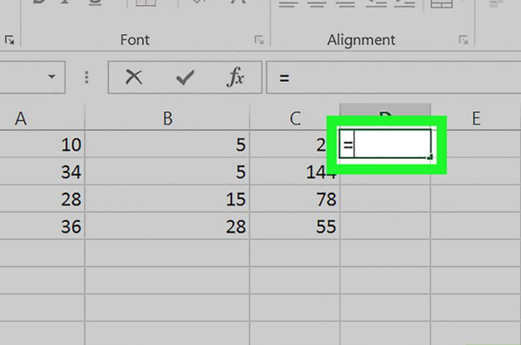With the cell selected, type in an equals symbol “=” into the cell. Type the value without the quotes. You will always need to type "equals" before writing the formulas in Excel. This basically tells Excel that you want to start writing a formula. You can think of it as a reverse math equation. We add the equals symbol at the end of math equations where we want the answers to appear in.

### Step Six: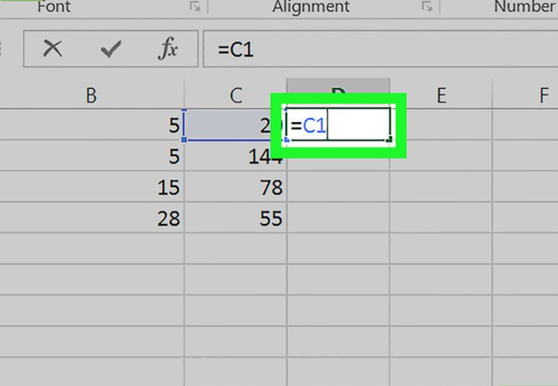Type a cell name. This is also called the cell reference in formulas. The cell whose name you type must be a cell from which you want to subtract the value or values of a cell or other cells.

For example, you could type "C1" to specify the number in cell C1.

### Step Seven: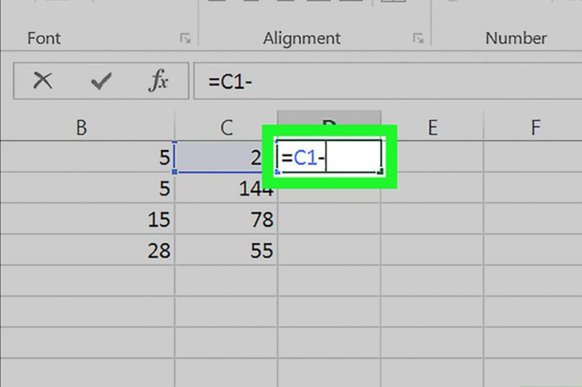Type in the dash or minus symbol “-“ after the first cell reference.

### Step Eight: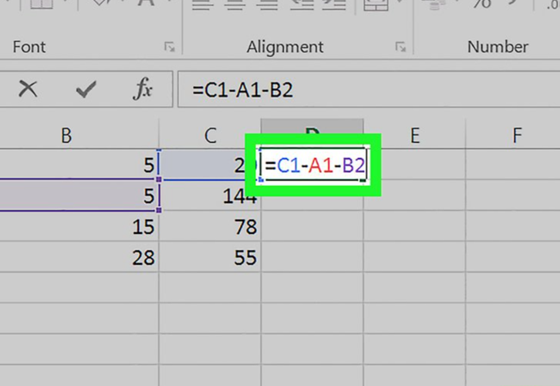Type the name of the other cells. This cell must be the cell you want to subtract from the first cell.

You can repeat this process with multiple cells (eg "C1-A1-B2").

### Step Nine: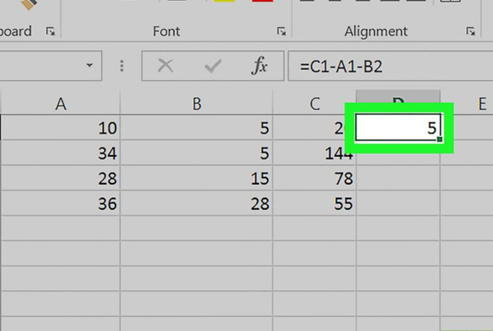Press ↵ Enter or ⏎ Return. Doing so will calculate the formula entered in the cell and replace it with the solution.

You can click on the cell to display the original formula in the formula bar above the row of characters. To edit the formula you’ve just made, you can double click on the cell or click on the formula bar. You can also use the keyboard shortcut F2.

## The Second Way: Subtraction within a cell

### Step One: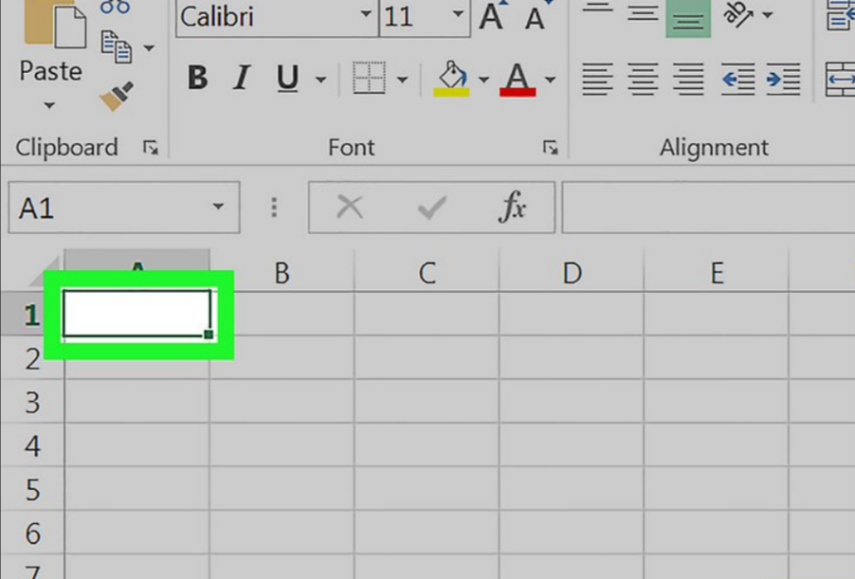Click on a cell. The cell you choose is not important unless you plan to create data with this workbook.

### Step Two: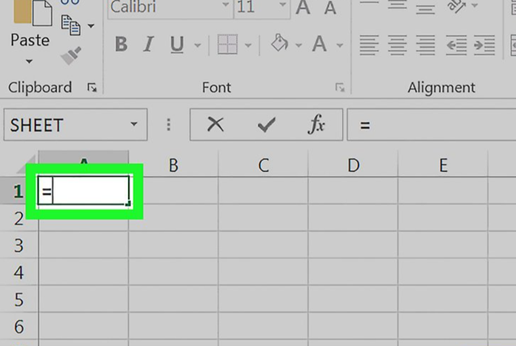Type “=” into the cell. Type the value without the quotes. This will prepare the cell for the formula.

### Step Three: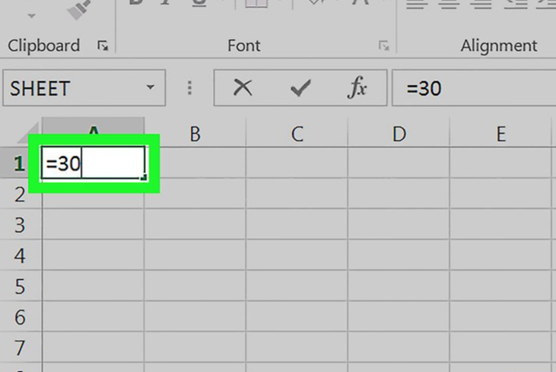Type the number you want to subtract from. The value will appear to the right of the equals sign in the selected cell.

For example, to set up your budget, you could type your monthly income into this cell.

### Step Four: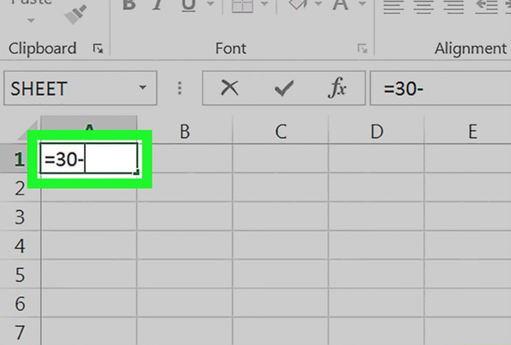Type (-) into the cell. The value will appear after the number you entered.

If you decide to subtract multiple values (X-Y-Z, for example), this step must be repeated after each successive number except for the last number.

### Step Five :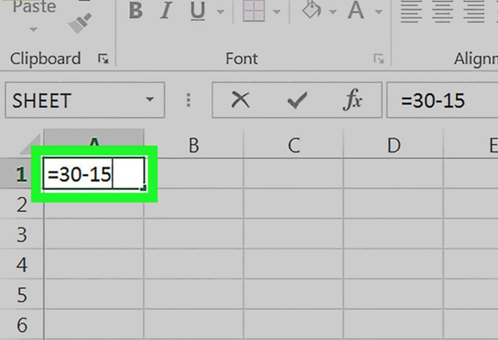Type the number you want to subtract from the first number.

For example, if you calculate a budget, you can write expenses in this cell.

Step Six: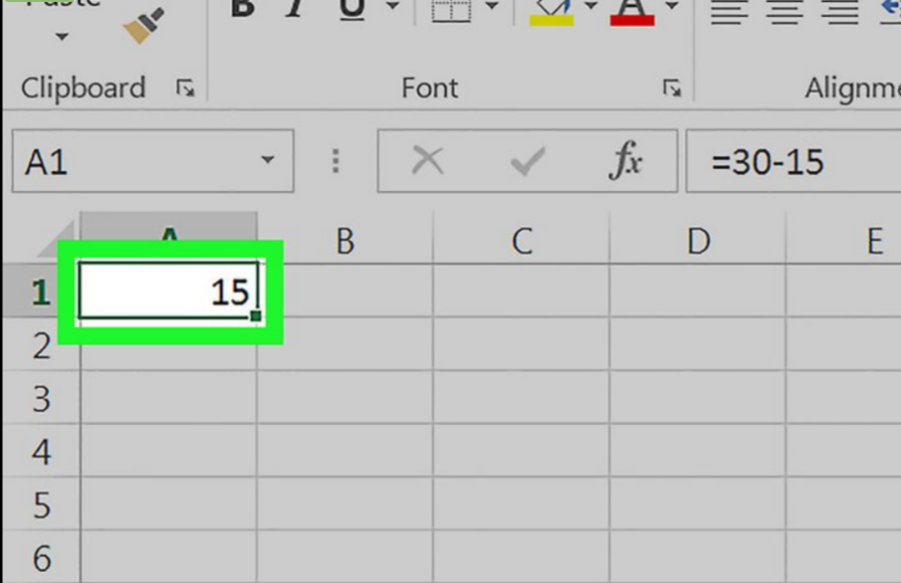Press ↵ Enter or ⏎ Return. Doing so will calculate the formula entered in the cell and replace it with the solution.

You can click on the cell to display the original formula in the text bar above the row of characters.

## The Third Way: Column Subtract

### Step One: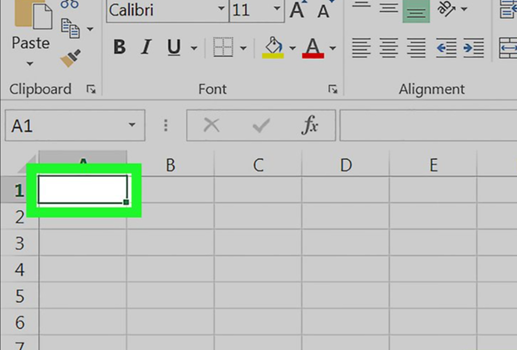Click on a cell. The cell you choose is not important unless you plan to create data with this workbook.

### Step Two: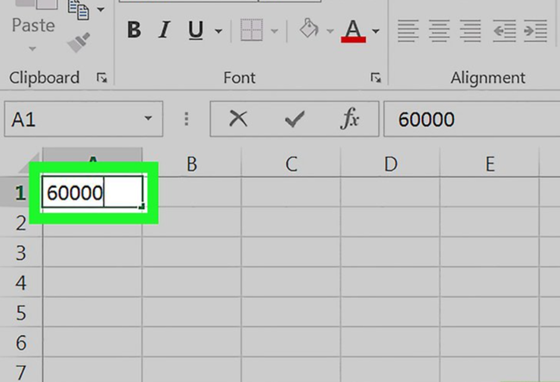Enter the key value. This is the number from which the rest of the column will be selected.

For example, you can write the annual salary in this field.

### Step Three: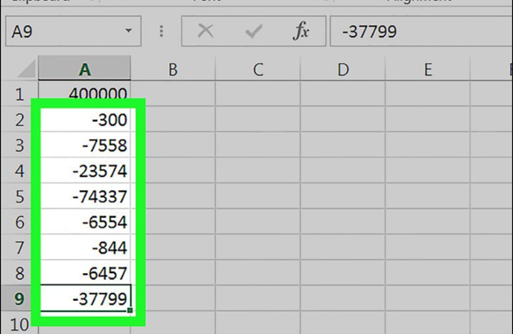Enter the deductions or the values you will be subtracting from the main value in the lower cells. To do this, type a negative value for the number you want to subtract (eg "-300" if you want to subtract 300).

• One value should be written for each cell.
• Make sure that every number you type is in the same column as the main number.
• To apply this to the salary example, you'd probably type (-) followed by an expense in each cell.

### Step Four: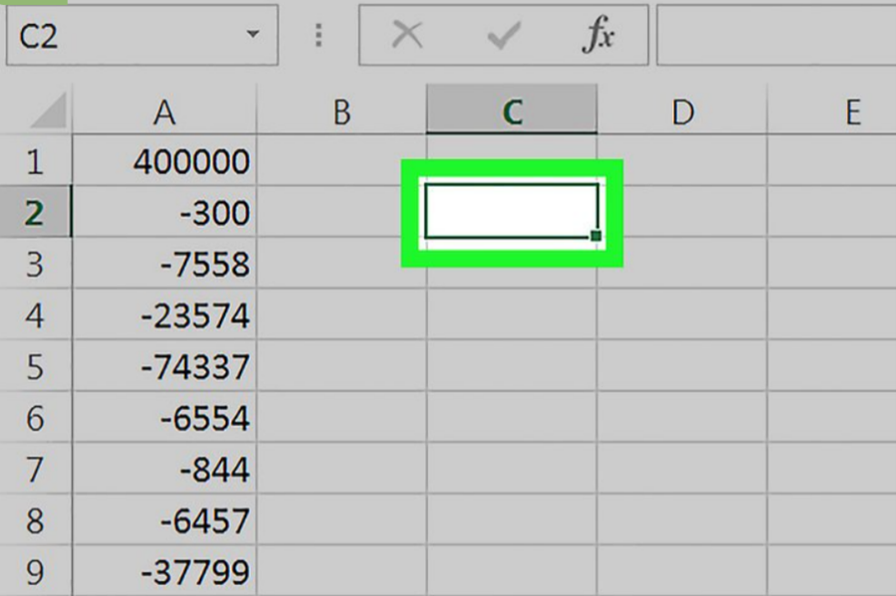Click on an empty cell. The cell does not need to be in the same column as the main value this time.

### Step Five: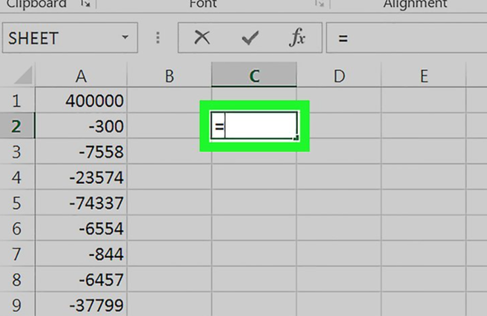Type “=” into the cell. Type the value without the quotes. Doing so prepares the cell for writing the formula.

### Step Six: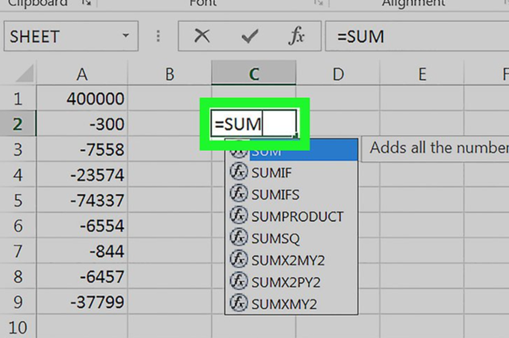Type SUM into the cell. The SUM command adds items together.

There is no direct "subtract" command, which is why numbers are entered in a negative form.

### Step Seven: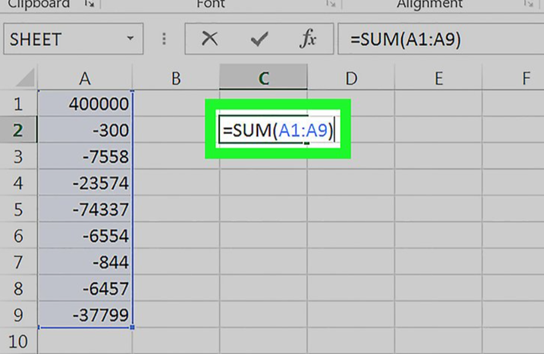Type (CellName:CellName) after SUM. This command adds all cells in a column from the value of the first cell to the value of the last cell.

For example, if cell K1 is the base number and the cell with the last value is cell 'K10', you should type '(K1:K10)'.

### Step Eight: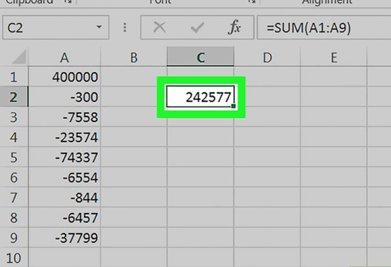Press ↵ Enter or ⏎ Return. Doing so will execute the formula in the selected cell, which will overwrite the formula with the final result.

## Some Quick Excel Tips

You can type formulas in Excel directly into a cell or the formula bar. You can also order formulas in Excel directly into a cell using functions. Dates, times, and percentages can also be calculated by entering formulas in Excel. Columns in Excel are denoted by letters such as A, B., C, etc. Rows in Excel are numbered 1, 2, 3, etc. When you intersect a column and a description, you get a cell address in Excel, which is indicated by the column letter and row number; for example, the first cell will be set as A1. You can also sign up for Simple Sheets website and get unlimited Excel ready-to-use templets.  You can also See Excel Functions.

Lorem ipsum dolor sit amet, consectetur adipiscing elit. Cras sed sapien quam. Sed dapibus est id enim facilisis, at posuere turpis adipiscing. Quisque sit amet dui dui.

#### Stay connected with news and updates!

Join our mailing list to receive the latest news and updates from our team.
Don't worry, your information will not be shared.

We hate SPAM. We will never sell your information, for any reason.